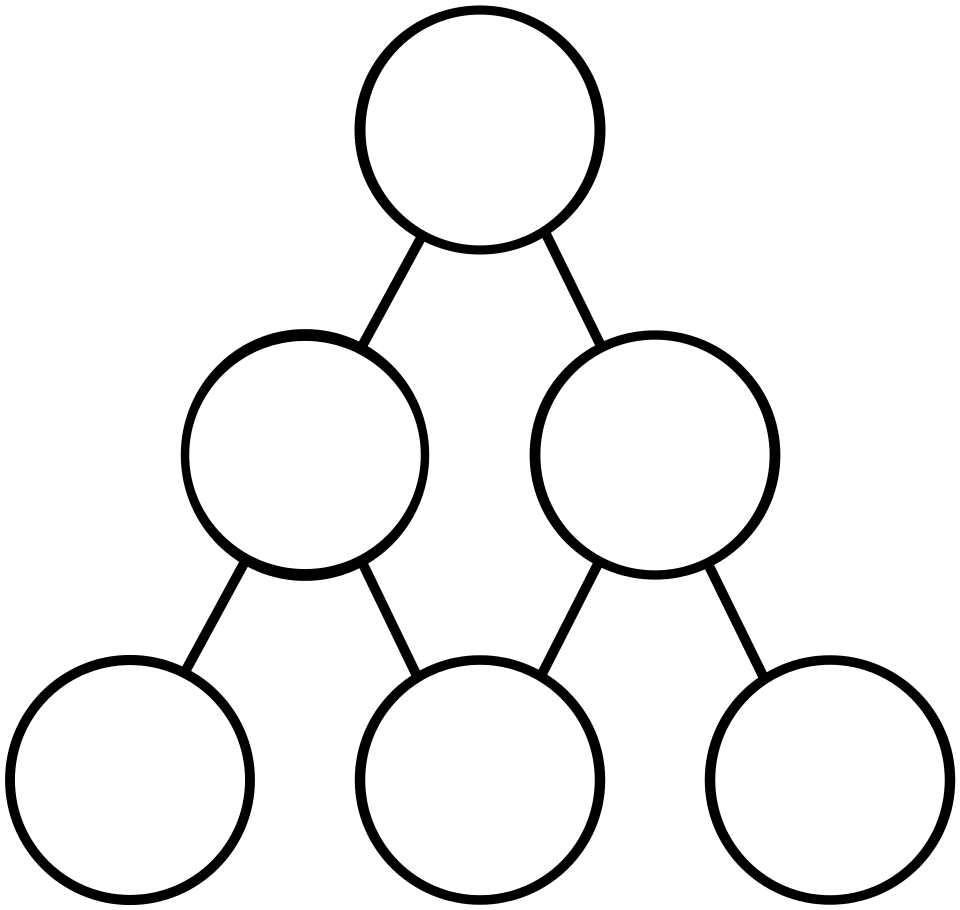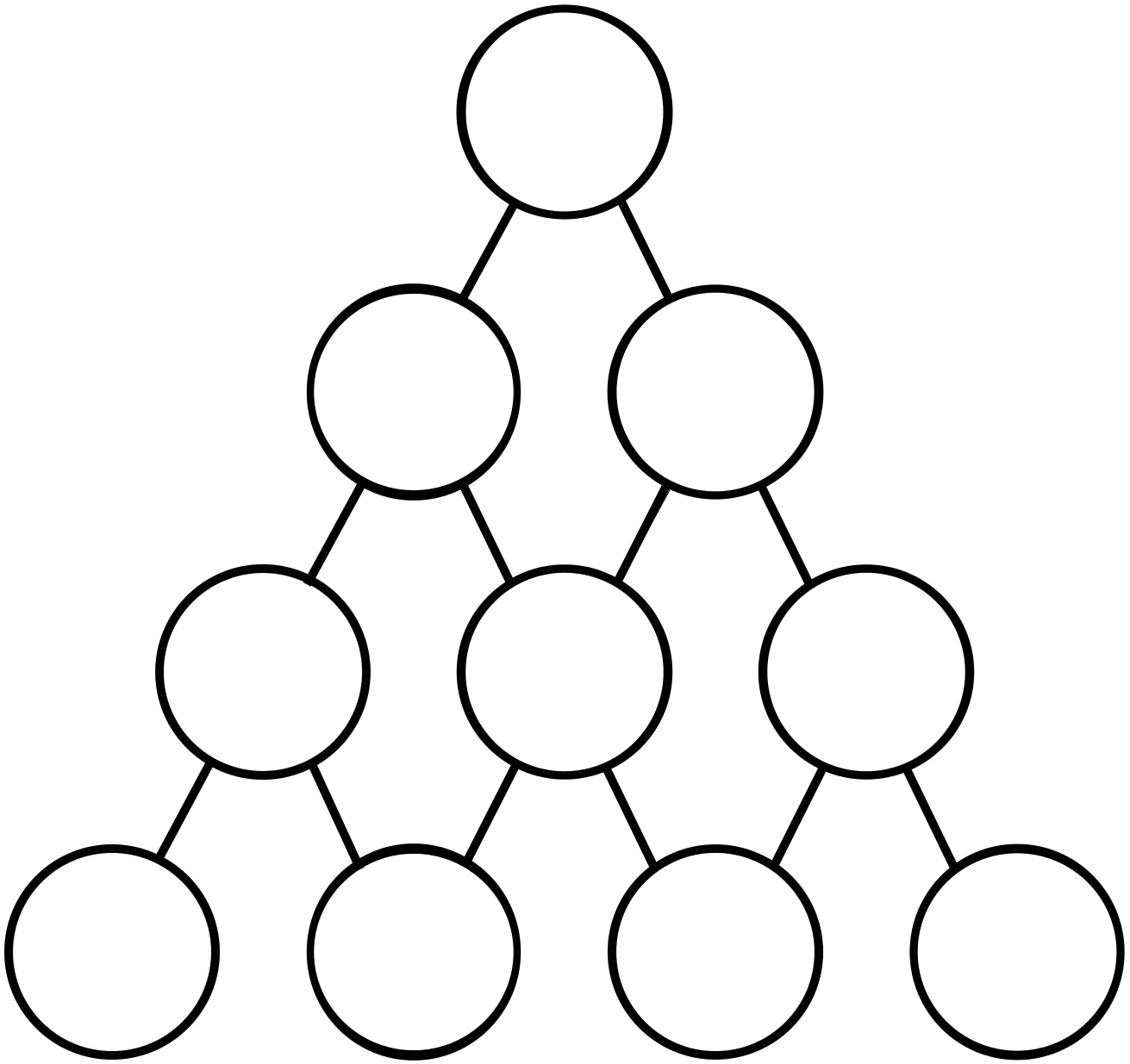#### You may also like### Matter of Scale

Can you prove Pythagoras' Theorem using enlargements and scale factors?### Overlap

A red square and a blue square overlap. Is the area of the overlap always the same?

# Positive Differences

##### Age 11 to 16Challenge Level

In the diagram below the number in each circle is the (positive) difference of the numbers in the two circles connected to it below.

Is it possible to to fill in the circles with the numbers $1$ to $6$, using each number exactly once?Can you show that:

• $6$ must be in the bottom row?
• $5$ cannot be at the top?
• $4$ cannot be at the top?

Can you find all of the possible solutions?

Extension

Can you fill these circles with the numbers $1$ to $10?$
Can you find all of the possible solutions?With thanks to Don Steward, whose ideas formed the basis of this problem.